# Lectures 8 9 Spectral Graph Theory February 3

• Slides: 17
Download presentationLectures 8 & 9 Spectral Graph Theory February 3, 2009 Monojit Choudhury [email protected] comSpectral Graph Theory Systematic study of the Eigenvalues and Eigenvectors of the adjacency matrix or the corresponding Laplacian matrix of the graph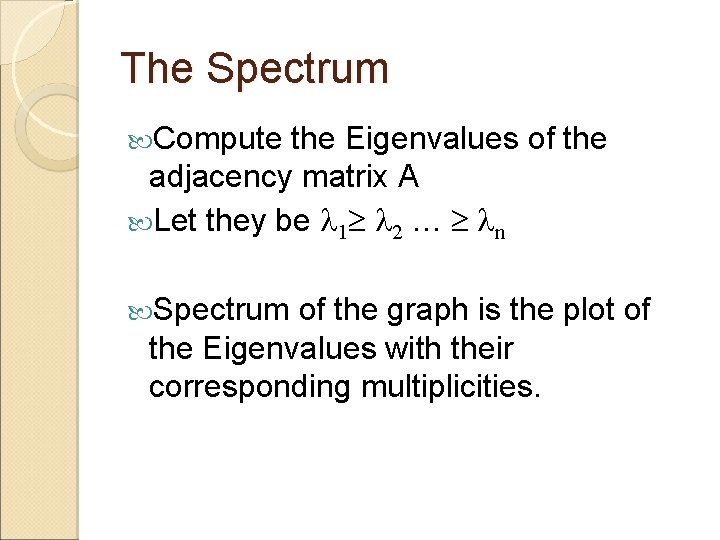The Spectrum Compute the Eigenvalues of the adjacency matrix A Let they be 1 2 … n Spectrum of the graph is the plot of the Eigenvalues with their corresponding multiplicities.Examples Find out the eigenvalues and eigenvectors for a triangle Can you generalize for an n-clique? What about a complete bipartite network?Banerjee and Jost (2007) Spectral Plots and Representation and Interpretation of Biological Data Spectral Plots of Random. Networks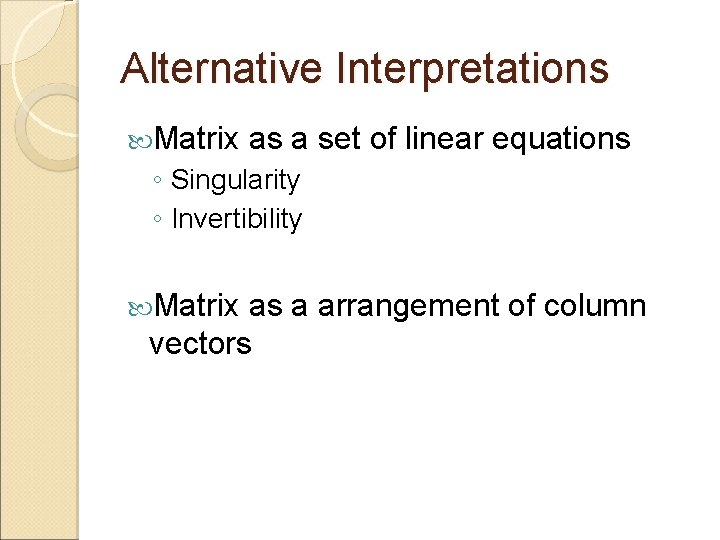Alternative Interpretations Matrix as a set of linear equations ◦ Singularity ◦ Invertibility Matrix as a arrangement of column vectors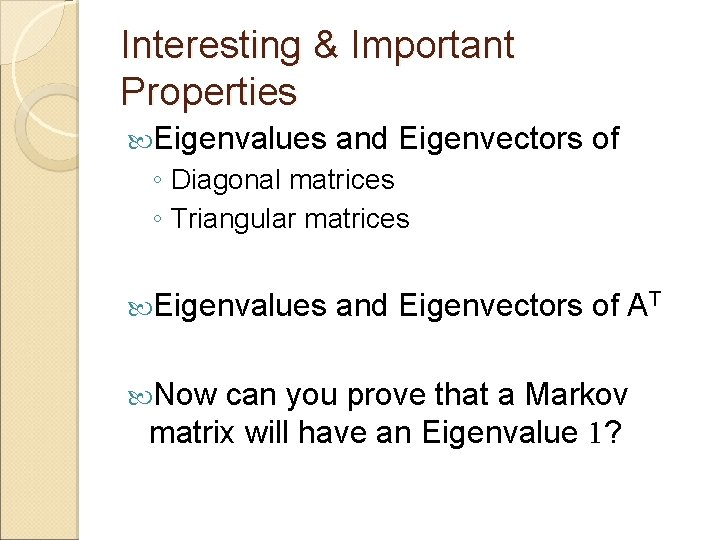Interesting & Important Properties Eigenvalues and Eigenvectors of ◦ Diagonal matrices ◦ Triangular matrices Eigenvalues Now and Eigenvectors of AT can you prove that a Markov matrix will have an Eigenvalue 1?More properties of Eigenvalues Trace of Matrix is the sum of the Eigenvalues Diagonalization of Matrices ◦ S-1 AS = Frobenius norm of symmetric matrices ◦ Spectral coverage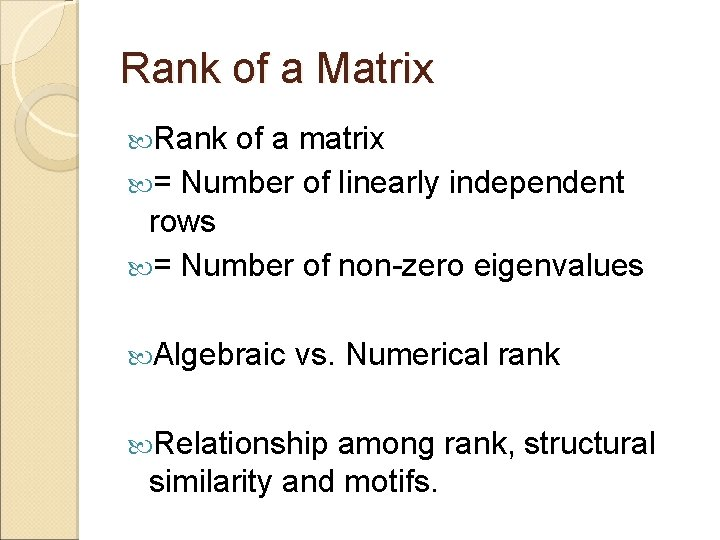Rank of a Matrix Rank of a matrix = Number of linearly independent rows = Number of non-zero eigenvalues Algebraic vs. Numerical rank Relationship among rank, structural similarity and motifs.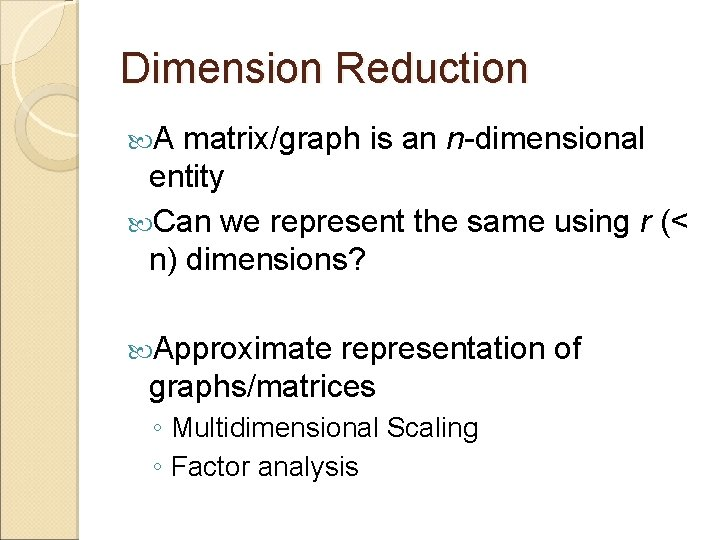Dimension Reduction A matrix/graph is an n-dimensional entity Can we represent the same using r (< n) dimensions? Approximate representation of graphs/matrices ◦ Multidimensional Scaling ◦ Factor analysis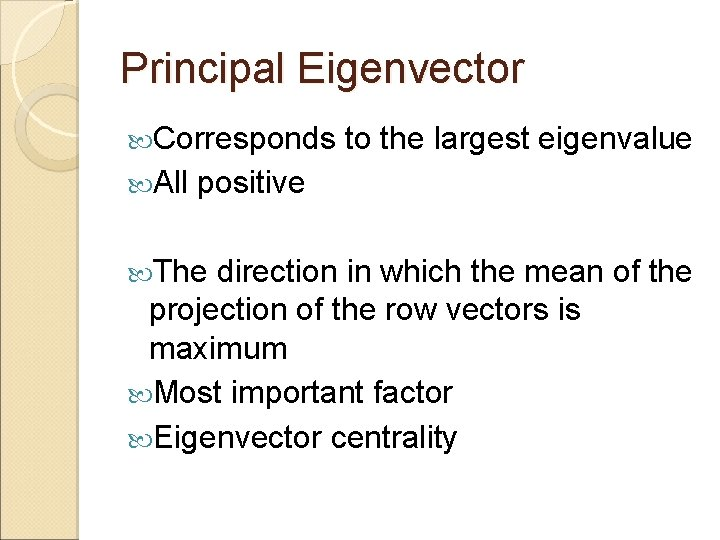Principal Eigenvector Corresponds All to the largest eigenvalue positive The direction in which the mean of the projection of the row vectors is maximum Most important factor Eigenvector centralityThe second eigenvector Always orthogonal to the principal eigenvector Used for spectral clustering Random walk based clustering ◦ Chinese whispers (Biemann, 2005) ◦ Affinity Propagation (Frey and Dueck, 2007)A case study (Mukherjee et al. 2009) /s/ l 2 l 1 /p/ 1 1 3 1 l 3 One-mode projection l 1 l 2 l 4 One-mode projection /t/ 1 l 4 1 1 /k/ l 3 2 1 2 1 1 1 2 /t/ /d/ /n/ /d/ 1 2 1 /p/ /n/ Lang. Graph B′ l 1 0 l 2 1 l 3 1 Pho. Net Pla. Net l 4 1 ATA – D′ A l 1 l 2 l 3 l 4 B /s/ /p/ /k/ /t/ /d/ /n/ /s/ 1 0 /s/ 0 0 1 /p/ 0 0 2 2 1 1 /k/ 1 1 0 1 /k/ 1 2 0 2 1 1 AAT – D l 2 1 0 1 3 l 3 1 1 0 2 /t/ 0 1 1 1 /t/ 0 2 2 0 1 2 l 4 1 3 2 0 /d/ 0 0 0 1 /d/ 0 1 1 1 0 1 /n/ 0 0 1 1 /n/ 1 1 1 2 1 0The Spectrum Eigenvalues Power-law exponent: -1. 33 Bulk of eigenvalues concentrated around zero Index of sorted eigenvalues Eigenvalues Power-law exponent: -0. 88 Index of sorted eigenvaluesThe spectral coverage 1 st Eigenvector: 89% 2 nd Eigenvector: 92% 3 rd Eigenvector: 93%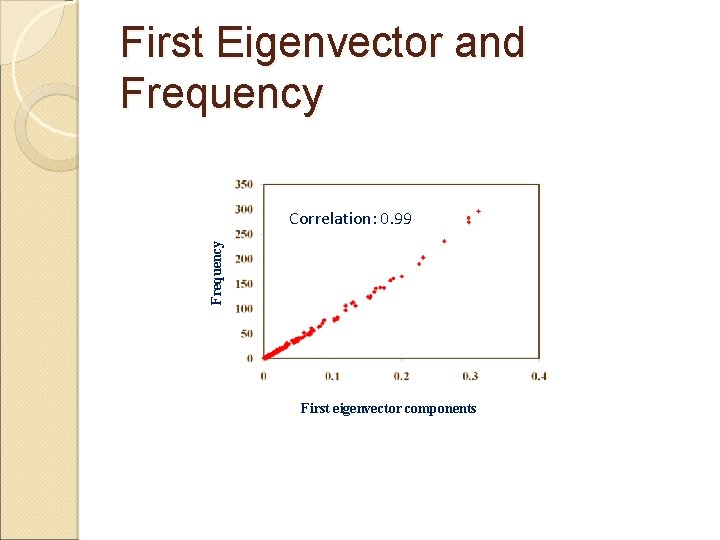First Eigenvector and Frequency Correlation: 0. 99 First eigenvector components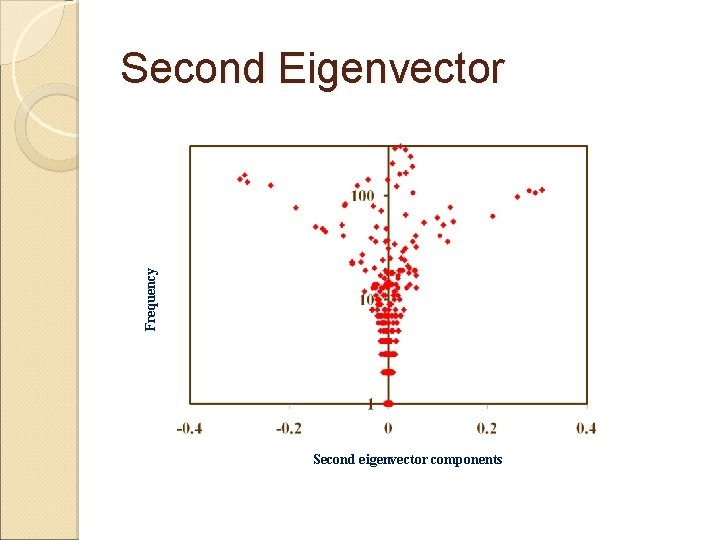Frequency Second Eigenvector Second eigenvector components